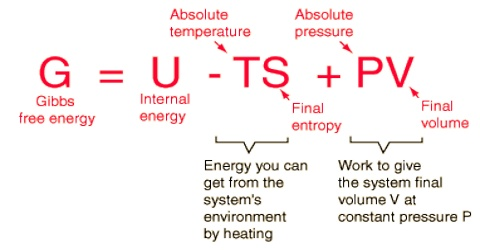Chemistry

# Gibbs Free Energy: Spontaneity and EquilibriumGibbs free energy, spontaneity and equilibrium

We know that useful work can be obtained from spontaneous natural processes. Since the free energy change is a measure of the useful work that might be obtained from a constant pressure process, one deduces the very important result that the decrease in free energy of a constant pressure process is the measure of the tendency of the process to proceed spontaneously. In other words, spontaneous processes are accompanied by decrease in free energy. Spontaneous processes can continue to occur as long as the free energy of the system can decrease, that is, until the free energy of the system reaches a minimum value. As in the equilibrium state the system has no further tendency to change, the system at equilibrium has a minimum value of the free energy; at equilibrium the system must satisfy the condition

dG = 0,

or, ∆G = 0If a process tends to be accompanied by an increase in free energy, the process will not proceed spontaneously in the direction considered. For constant pressure processes the relations of free energy change to spontaneity and equilibrium may be summarized as follows:

• ∆G < 0 (negative), the process is spontaneous
• ∆G > 0 (positive); the process is non-spontaneous
• ∆G = 0; the system is at equilibrium.

It has to be pointed out that in these statements no mention is made of the surroundings and the free energy change is that for the system only. Thus the inconvenience of the entropy criterion at equilibrium has been eliminated.